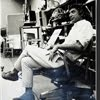## Eugene Ray SDSU 1970'sEugene Ray 1970's. photo credit: Tom Davis

## Saturday, November 24, 2012

### EUGENE RAY ARTIST=ARCHITECT =PROFESSOR is how my oeuvre title should read as a COMPLETE survey contains LARGE VARIETY ! ^^^^^^^^^^^^^^^^^^^^^ CATALOG OF WORK IS NEEDED> to list all of my creative output> ^^^^^^^^^^^^^^^^^^^^^ OEUVRE ENCOMPASES A LARGE AMOUNT OF METAPHYSIC ART ! architecture & metaphysic work are symbiotic in overall catalog> ^^^^^^^^^^^^^^^^^^^^^ 5 PHOTOS ARE=INTRODUCTION ! Inbox+EUGENE RAY+
+ARTIST=ARCHITECT+
+PROFESSOR+
^^^^^^^
^^^^^^^^^^^^^^

+RECENT QUESTION+
>just how many buildings have<
>you constructed anyway< ?
^^^^^^^^^^^^^
+IS A QUESTION THAT+
>(i don't know)<
+MISSES THE POINT+
^^^^^^^^^^
>as an artist-architect (and professor)<
>my oeuvre (complete work) by its<
+N A T U R E+
^^^^^^
+CONTAINS UNBUILT PROTOTYPES+
>that are extremly important<
>to the future of earthly<
(>and non-earthly<)
^^^^^^^^^
+ARCHITECTURAL HISTORY+
^^^^^^^^^^^^
>especially biotronic, biomorphic, and<
>tensegrity experimental prototypes<
+STORM RESISTANT+
>that the architectural profession<
>has no time (\$\$\$) to pursue<
^^^^^^^^^^^^^^^
+ALSO I GAVE MANY DESIGNS+
+AWAY W/O KNOWLEDGE+
>(far off places)<
+OF THEIR OUTCOME+
^^^^^^^^^^
>pedagogical (university) position<
(+IN MY EYES+)
>encouraged my philanthropy<
(>i am not very generous<
>in normal activities<)
^^^^^^^^^

+TONIGHT'S PHOTOS+
>reveals important prototypes that<
>offer much to the progress of<
+ARCHITECTURE+
^^^^^^^^
(>dirk sutro in his kind article about<
+LOS ANGELES TIMES+
>my work described the fact i had<
>a construction history besides<
>the visionary prototypes<)
^^^^^^^^^^^^^

#1)          +OEUVRE CATALOG+
>should contain all visionary designs<
>many which are more important<
>then the constructed oeuvre<
^^^^^^^^^^^^^^^
+WRIGHT=GOFF=CORBUSIER+
+LEONARDO DA VINCI+
>best work is in this category<
>study this very carefully<
^^^^^^^^^^^^^
+LEONARDO'S FORTRESS DESIGN+
(>in my estimation<)
+EQUAL TO THE MONA LISA+
^^^^^^^^^^^^^^

#2)            +DOUBLE DOME+
>prototype is extremly important as<
+STORM-EARTHQUAKE+
>proof design=aerodynamic and<
+RAISED 10 FEET+
^^^^^^^^^^^^^^^^
+PERFECT SOLUTION TO "KATRINA"+
+& "SANDY" STORM THREATS+
^^^^^^^^^^^^^^^^
(>domes have superior strength<
>plus the biotronic vitality<)
^^^^^^^^^^^^^^

#3)       +DOCUMENTA 1980+
>retospective review of my design work<
>after 10 years in california did show<
>the essence of creative output<
+INCLUDING+
>synergetic environ, furniture and<
>design communication inventions<
^^^^^^^^^^^^^^^^^^^
>are evident and increasingly vital<
>the images here were selected<
>with much detailed care<
^^^^^^^^^^^^^

>plantation prototype for<
+LONGER LIFE 1982+
^^^^^^^^^
>is one of my favorite all-time design<
>concepts for a modern prototype<
+FOR 3 GENERATIONS OF+
+THE SAME FAMILY+
(>organic, close to the land, life<
^^^^^^^^^^^

#5)      +HEALING SYNERGETICS+
>describes the solar heated and wind<
>energised prototype designed for<
+SELF SUFFICIENT+
>active, healthy, daily activity to<
>support a vinyard plantation<
^^^^^^^^^^^^^^^

+GENETIC MEMORY+
>of my ancestors plantation life is<
>no doubt a factor here but<
^^^^^^^^^^^^^^^
+THE WHOLE EARTH CATALOG+
>vision for living<
+IS ALSO VERY IMPORTANT+
^^^^^^^^^^^^^
+NOTE+
(>shall cover the metaphysical<
+C O L L A G E+
>graphics in the future<)
^^^^^^^^^^^

gene ray, lajolla, california

>eugene ray, mfa, architect<
>professor emeritus, sdsu<